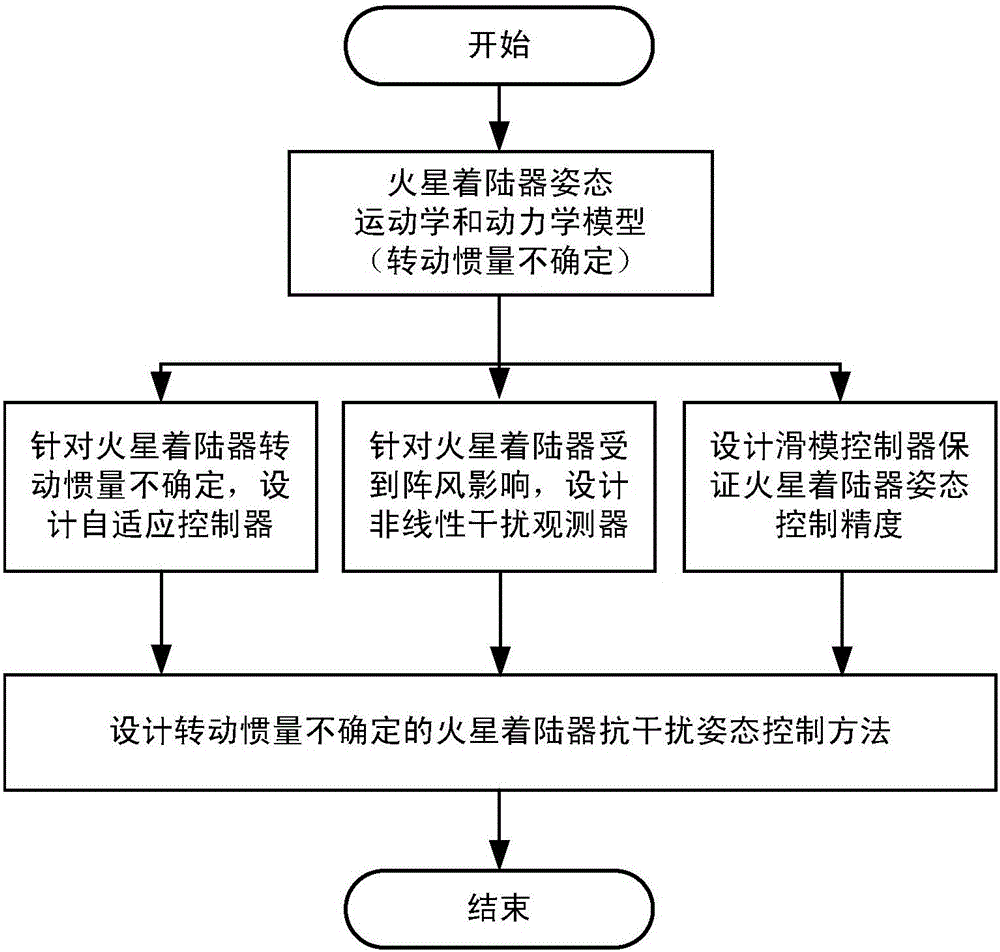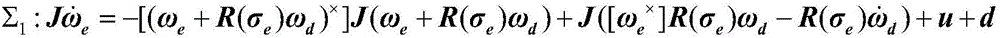# Anti-interference attitude control method for Mars lander with uncertain rotational inertia

## A technology of moment of inertia and attitude control, applied in the direction of the guidance device of space navigation vehicle, can solve the problem of inability to guarantee the attitude control accuracy of the lander, and achieve the effect of improving engineering application value, anti-interference and adaptability

Active Publication Date: 2016-09-21
BEIHANG UNIV
4 Cites 1 Cited by

## AI-Extracted Technical Summary

### Problems solved by technology

However, the traditional attitude control method mainly considers the accuracy of the attitude control system, and ignores the uncertain moment of inertia and gusts that the Mars lander will face during flight. As a result, the attitude control accu...
View more

## Abstract

The invention relates to an anti-interference attitude control method for a Mars lander with uncertain rotational inertia. The anti-interference attitude control method comprises the steps that firstly, an attitude kinematics and dynamics model of the Mars lander with the uncertain rotational inertia is established; secondly, aiming at the influence brought by the uncertain rotational inertia of the Mars lander, a self-adaptive controller is designed to estimate the uncertain rotational inertia; thirdly, the interference influence of gust on the Mars lander is estimated through a nonlinear interference observer, and then the gust estimated value is fed back to a control system to be subjected to feedforward offset; and finally, the nonlinear interference observer, the self-adaptive controller and a sliding mode controller for ensuring the system attitude precision are composited, so that the anti-interference attitude control method for the Mars lander with the uncertain rotational inertia is constructed. The anti-interference attitude control method for the Mars lander with the uncertain rotational inertia has the characteristics of being high in anti-interference performance and adaptability, and compared with a traditional Mars lander attitude control method, the practical engineering value is higher.

Application Domain

Spacecraft guiding apparatus

Technology Topic

## Image

•••## Examples

• Experimental program(1)

### Example Embodiment

 Such as figure 1 As shown, the specific implementation steps of the present invention are as follows (the following takes the Mars lander attitude control system as an example to illustrate the specific implementation of the method):
 1. Build the attitude kinematics and dynamics model of the Mars lander with uncertain moment of inertia
 The posture kinematics and dynamics model of Mars lander with uncertain moment of inertia is constructed as follows:
 X 1 : J ω · e = - [ ( ω e + R ( σ e ) ω d ) X ] J ( ω e + R ( σ e ) ω d ) + J ( [ ω e X ] R ( σ e ) ω d - R ( σ e ) ω · d ) + u + d
 X 2 : σ · e = G ( σ e ) ω e
 X 3 : G ( σ e ) = 1 2 ( 1 - σ e T σ e 2 I 3 + σ e σ e T - [ σ e X ] )
 X 4 : J = J 11 J 12 J 13 J 12 J twenty two J twenty three J 13 J twenty three J 33
 X 5 : [ ω e X ] = 0 - ω e z ω e y ω e z 0 - ω e x - ω e y ω e x 0
 Among them, J represents the moment of inertia of the Mars lander in the body coordinate system, such as Σ 4 As shown, the value is It is an unknown parameter when designing the controller. ω e =[ω ex ,ω ey ,ω ez ] T Indicates the tracking error of the three-axis angular velocity of the Mars lander, σ e =[σ ex ,σ ey ,σ ez ] T Indicates the three-axis modified Rodrigues parameter (ModifiedRodrigues Parameter, MRP) tracking error of the Mars lander. ω d =[ω x ,ω y ,ω z ] T Indicates the desired angular velocity of the three axes of the Mars lander, where the initial attitude MRP value and the initial angular velocity are respectively σ 0 =[-0.169,-0.014,0.526] T And ω 0 =[0,0,0] T , The expected attitude MRP value and the expected angular velocity are respectively σ d =[0.131 0.186 0.226] T with u=[u x ,u y ,u z ] T Represents the three-axis control input of the Mars lander. d=[d x ,d y ,d z ] T Indicates the interference torque of the three-axis gust to the Mars lander, and assume that the interference torque is
 2. Construct an adaptive controller
 Aiming at the uncertain moment of inertia in the attitude kinematics and dynamics model of the Mars lander, an adaptive controller is constructed to estimate the moment of inertia.
 First define the linear operator L(χ), the specific form is as follows:
 X 6 : L ( χ ) = χ 1 χ 2 χ 3 0 0 0 0 χ 1 0 χ 2 χ 3 0 0 0 χ 1 0 χ 2 χ 3
 Then there are:
 X 7 :Jχ=L(χ)θ
 Where θ=[J 11 J 12 J 13 J 22 J 23 J 33 ] T ∈R 6 Is the parameter vector to be identified.
 The estimated value of the moment of inertia J is And transform the uncertainty of the estimated moment of inertia into the uncertainty of the estimated θ, and the estimated value is
 Design the adaptive control law as follows:
 X 8 : u 1 = - k W θ ^ θ ^ · = Γ W T F s
 Where k is a scalar greater than 0,
 X 9 : s = σ · + k σ
 X 10 : W = ( F σ ) X L ( ω ) + L ( ω - F G · ( σ e ) F σ )
 Among them, W is an auxiliary variable, F=G(σ e ) -1 , S is the sliding surface.
 3. Design a nonlinear interference observer
 In order to facilitate the design of the nonlinear disturbance observer, the system Σ 1 , Σ 2 Converted to type such as Σ 11 The nonlinear system model:
 X 11 : x · ( t ) = f ( x ( t ) ) + g 1 ( x ( t ) ) u + g 2 ( x ( t ) ) d y ( t ) = h ( x ( t ) )
 x ∈ R 6 Is the state vector, u∈R 6 Is the control input, d∈R 3 Is the interference torque. among them,
 f ( x ( t ) ) = J - 1 ( - [ ( ω e + R ( σ e ) ω d ) X ] J ( ω e + R ( σ e ) ω d ) + J ( [ ω e X ] R ( σ e ) ω d - R ( σ e ) ω · d ) ) G ( σ e ) ω e ,
 R ( σ e ) = [ ( 1 - 6 σ e 2 + σ e 4 ) I 3 + 8 σ e σ e T - 4 ( 1 - σ e 2 ) [ σ e X ] ] ( 1 - σ e 2 ) 2 , g 1 ( x ( t ) ) = g 2 ( x ( t ) ) = J - 1 0 ,
 h(x(t))=x(t).
 Assume that the interference model is like Σ 12 :
 X 12 : ξ · = A ξ d = C ξ
 ξ∈R 6×6 , D∈R 3 , The disturbance d is continuously applied to the system Σ 11 on.
 Design disturbance observer Σ 13 :
 X 13 : z · = ( A - l ( x ) g 2 ( x ) C ) z + A p ( x ) - l ( x ) ( g 2 ( x ) C p ( x ) + f ( x ) + g 1 ( x ) u ) ξ ^ = z + p ( x ) d ^ = C ξ ^
 Where z∈R 6×6 Is the state variable of the observer, p(x)∈R 6×6 Is a non-linear function of the design. The gain of the nonlinear disturbance observer is Σ 14 formula:
 X 14 : l ( x ) = ∂ p ( x ) ∂ x
 4. Combine the nonlinear interference observer, the adaptive controller and the sliding mode controller to ensure the system attitude accuracy to construct an anti-jamming attitude control method for the Mars lander with uncertain moment of inertia
 First, design the sliding mode controller to ensure the attitude accuracy as follows:
 X 15 :u 2 =-G T (σ e )k s sgn(s)-G T (σ e )gs
 Where k s And g are three-dimensional diagonal positive definite matrix.
 Secondly, the form of the composite controller is constructed as follows:
 X 16 : u = u 2 + u 1 - d ^ .
 The invention has the characteristics of strong anti-interference and strong adaptability, and has higher engineering practical value than the traditional Mars lander attitude control method.
 The content not described in detail in the specification of the present invention belongs to the prior art known to those skilled in the art.

## PUM## Description & Claims & Application Information

We can also present the details of the Description, Claims and Application information to help users get a comprehensive understanding of the technical details of the patent, such as background art, summary of invention, brief description of drawings, description of embodiments, and other original content. On the other hand, users can also determine the specific scope of protection of the technology through the list of claims; as well as understand the changes in the life cycle of the technology with the presentation of the patent timeline. Login to view more.

## Device testing system and method

ActiveCN109856521AHigh engineering application value
Owner:北京京能新能源有限公司

## DVL calibration method for ignoring horizontal attitude of AUV integrated navigation system

PendingCN114440925AHigh positioning accuracyHigh engineering application value
Owner:宜昌测试技术研究所

## Signal detection method of speed sensor based on eddy current principle

Owner:TIANJIN JINHANG COMP TECH RES INST

PendingCN106912192AHigh engineering application valueSimple structure
Owner:NANJING UNIV OF INFORMATION SCI & TECH

## Direct-driven wind turbine group simulation model and data acquisition method and system thereof

InactiveCN111884257AReduce the amount of simulation calculationHigh engineering application value
Owner:NORTH CHINA ELECTRICAL POWER RES INST +2

## Signal processing method based on quasi high-order square spectrum

ActiveCN109547371AReduce computational complexityHigh engineering application value
Owner:XIDIAN UNIV

## Classification and recommendation of technical efficacy words

• High engineering application value